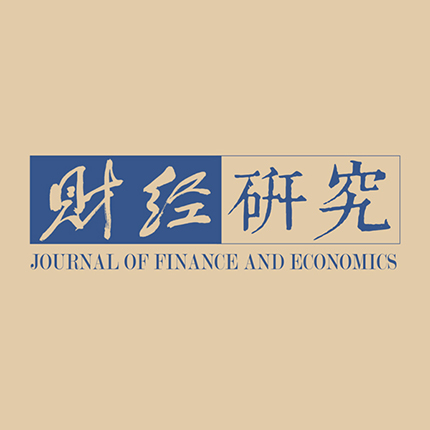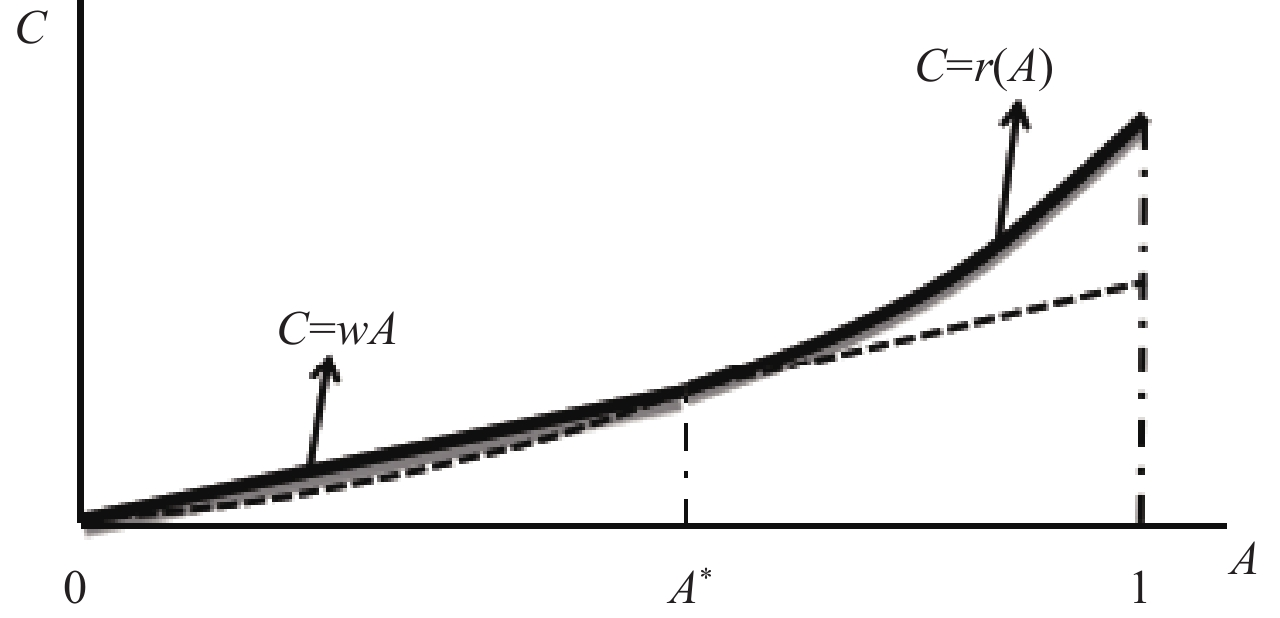﻿ 债务扩张、劳动力职业选择与人力资本配置《财经研究》
2023第49卷第5期

1. 上海大学 经济学院, 上海 201900;
2. 南开大学 经济学院, 天津 300071

Debt Expansion, Labor Occupation Choice and Human Capital Allocation
Shao Wenbo1, Li Rui1, Shi Bingzhan2
1. School of Economics, Shanghai University, Shanghai 201900, China;
2. School of Economics, Nankai University, Tianjin 300071, China
Summary: In recent years, a large number of highly skilled workers have poured into the public sector and non-productive monopoly state-owned enterprises represented by financial institutions, resulting in a high concentration of human capital in these sectors. On the contrary, the physical sector represented by manufacturing industry has a talent shortage. This mismatch of human capital deserves special attention, especially in the critical period of China’s economic transformation from high-speed growth to high-quality development. To achieve the goal of building a powerful manufacturing country and advanced manufacturing industry, innovation is required, and talent strategy is fundamental. The existing studies are mainly carried out from the perspectives of labor remuneration and industrial monopoly, but there is one fact that cannot be explained: On the one hand, with the deepening of reform, the wage formulation of public sectors and state-owned enterprises is becoming more and more market-oriented, and monopoly and power rent-seeking are also gradually weakened; on the other hand, the labor occupation choice is biased towards these sectors, resulting in the increasingly serious problem of human capital mismatch. This paper concerns that the debt expansion of local governments based on land finance may be an important reason for the above problems. First, the model is used to prove that the debt expansion of local governments will expand the scale of public sectors and financial industry, and attract labor force through direct and indirect effects. Due to the existence of “entry threshold” in these sectors and the failure of compensation system marketization, the “siphon” effect of these sectors on highly skilled labor force has led to the mismatch of human capital with physical sectors. Second, using the data of China Family Panel Studies (CFPS) and dividing local government debt into explicit and implicit categories, it is found that with the debt expansion of local governments, the highly skilled labor force is more inclined to public sectors and financial industry in terms of the occupation choice, and will form a wage gap compared with physical sectors, resulting in human capital mismatch. Classifying all the industry into public sectors, state-owned enterprises and private enterprises, it is found that with the acceleration of the debt expansion of local governments, more skilled labor will be attracted to public sectors and state-owned enterprises, resulting in excess human capital. Further research also finds that the improvement of regional marketization can effectively curb the above effects. This conclusion, to some extent, explains the reason why the current occupation choice of labor force tends to public sectors, which provides new evidence for regulating and controlling local government debt, and expands the solution to the problem of human capital mismatch from different angles.
Key words: land finance    financial industry    occupation choice    human capital mismatch

（一）基准模型。假定劳动力的技能 $A\in \left[\mathrm{0,1}\right]$ ，服从密度函数为 $f\left(A\right)$ 的分布。全社会只有私人部门，不存在市场失灵等需要政府介入的因素。许多企业只生产一种产品，每个企业由一个技能为A的经理和总技能为H的工人组成，A大于任何一个单个工人的技能。生产函数为： $y=AF\left(H\right)$ ，其中y表示产出， $F\left(A\right)$ 是单调增加函数，并且 ${F}^{{'}}\left(\cdot \right) > 0,{F}^{''}\left(\cdot \right) < 0$ 。将产品的价格标准化为1，则经理的收入为：

 $r=AF\left(H\right)-wH$ (1)

 $H=H\left(A\right)={{F}^{{'}}}^{-1}(w/A)$ (2)

 ${\int }_{0}^{{A}^{*}}Af\left(A\right){\rm{d}}A={\int }_{{A}^{*}}^{1}H\left(A\right)f\left(A\right){\rm{d}}A$ (3)

 ${A}^{*}F\left(H\left({A}^{*}\right)\right)-wH\left({A}^{*}\right)=w{A}^{*}$ (4)

 $Y={\int }_{{A}^{*}}^{1}AF\left(H\right)f\left(A\right){\rm{d}}A$ (5)图 1 单个部门不同技能劳动力的职业选择和工资决定示意图

 $C=\left\{\begin{array}{c}wA，A\in [0,{A}^{*})\\ r\left(A\right)，A\in [{A}^{*},1]\end{array}\right.$ (6)

（二）加入政府的两部门模型。模型设定沿用传统经济学的基本假定，即政府只提供公共产品，并不提供实际产品。所以全社会仍然只有一种产品。因而政府部门的产出并不是具体的产品，而是维护市场和社会正常运行的治理水平。不同于基准模型中假定的理想的市场环境，现实中市场环境的正常运行需要政府进行治理。具体地，假定政府治理函数为 $G=G\left(A\right)$ ，是单调增加函数， ${G}^{{'}}\left(\cdot \right) > 0,{G}^{{''}}\left(\cdot \right) < 0$ 其中A为政府雇佣劳动力（以下称为公务员）的技能水平。鉴于政府部门所从事的是管理、协调和决策之类的工作，对于公务员的技能有一定的要求，这里假定 $A\ge {A}_{2}$ ${A}_{2}$ 表示公务员的最低技能要求，并且为分析方便假定 ${A}_{2}$ 不小于工人的最高技能 ${A}_{1}$

 $c\left(A\right)=\rho G\left(A\right)$ (7)

 \begin{aligned} \varPsi \left(A\right)&= \left(AF\left(H\right)-wH\right)\left(1-t\right)\left(1-q\right)-\rho G\left(A\right)\\ &=\left(AF\left(H\right)-wH\right)\left(1-t\right)\left(1-q\right)-\frac{t\int \left(AF\left(H\right)-wH\right)\left(1-q\right)f\left(A\right){\rm{d}}A}{\int G\left(A\right)f\left(A\right){\rm{d}}A}G\left(A\right)\\ &=R\left(A\right)\left(1-t\right)\left(1-q\right)-t\left(1-q\right)\frac{\int R\left(A\right)f\left(A\right){\rm{d}}A}{\int G\left(A\right)f\left(A\right){\rm{d}}A}G\left(A\right)\\ &=R\left(A\right)\left(1-t\right)\left(1-q\right)-t\left(1-q\right)\frac{\stackrel-{R}}{\stackrel-{G}}G\left(A\right) \end{aligned} (8)

 $\varPsi \left({A}_{2}\right)=R\left({A}_{2}\right)\left(1-t\right)\left(1-q\right)-t\left(1-q\right)\frac{\stackrel-{R}}{\stackrel-{G}}G\left({A}_{2}\right) < 0$ (9)

（9）式表示技能水平为 ${A}_{2}$ 的劳动力，当选择做经理的预期利润低于选择公务员获得的报酬时，正常情况下会进入政府部门。由此可得税率t的最小值。此后，随着政府规模的扩张，亦即随着t的增加，会有越来越多的高技能劳动力进入政府部门。

 $\left(1-t\right)\left(1-q\right)R\left({A}_{1}\right)=w{A}_{1}$ (10)

 $\left(1-t\right)\left(1-q\right)R\left({A}_{3}\right)=\rho G\left({A}_{3}\right)$ (11)

 ${\int }_{0}^{{A}_{1}}Af\left(A\right){\rm{d}}A={\int }_{{A}_{1}}^{{A}_{2}}H\left(A\right)f\left(A\right){\rm{d}}A+{\int }_{{A}_{3}}^{1}H\left(A\right)f\left(A\right){\rm{d}}A$ (12)

 $t\left(1-q\right)\left[{\int }_{{A}_{1}}^{{A}_{2}}R\left(A\right)f\left(A\right){\rm{d}}A+{\int }_{{A}_{3}}^{1}R\left(A\right)f\left(A\right){\rm{d}}A\right] = \rho \bar{G}$ (13)

 \begin{aligned} \; \\ C=\left\{\begin{array}{l}wA，A\in \left[0,{A}_{1}\right)\\ r\left(A\right)or 0，A\in \left[{A}_{1},{A}_{2}\right)\cup \left({A}_{3},1\right]\\ c\left(A\right)，A\in \left[{A}_{2},{A}_{3}\right]\end{array}\right. \end{aligned} (14)图 2 加入政府部门后不同技能劳动力的职业选择和工资决定示意图

 $\frac{\partial \varPsi }{\partial t}=-\left[\left(1-q\right)+{q}^{{'}}\left(1-t\right)\right]R\left(A\right)-(1-q-t{q}^{{'}})\frac{\stackrel-{R}}{\stackrel-{G}}G\left(A\right)$ (15)

 $E={\int }_{{A}_{1}}^{{A}_{2}}Af\left(A\right){\rm{d}}A+{\int }_{{A}_{3}}^{1}Af\left(A\right){\rm{d}}A$ (16)

 $P={\int }_{{A}_{2}}^{{A}_{3}}Af\left(A\right){\rm{d}}A$ (17)

 $N={\int }_{{A}_{2}}^{{A}_{3}}\left[\rho G\right(A)-(1-t\left)\right(1-q\left)R\right(A\left)\right]f\left(A\right){\rm{d}}A$ (18)

（一）模型设定。针对命题1，考虑到被解释变量为劳动力职业选择，使用Logit模型进行回归，借鉴Nunn（2007），使用地方政府债务与劳动力技能水平的交叉项作为解释变量，具体模型设定如下：

 \begin{aligned} \mathrm{Pr}\left({Choice}_{ict}=1\right)&=\mathrm{Pr}\left({\alpha }_{0}+{\alpha }_{1}{X}_{ict}+{\alpha }_{2}{X}_{ict}\times {H}_{ct}+{\alpha }_{i}{D}_{i}+{\alpha }_{c}{E}_{c}+{\mu }_{c}+{v}_{t}+{\varepsilon }_{ict} > 0\right)\\ &=F\left({\alpha }_{0}+{\alpha }_{1}{X}_{ict}+{\alpha }_{2}{X}_{ict}\times {H}_{ct}+{\alpha }_{i}{D}_{i}+{\alpha }_{c}{E}_{c}+{\mu }_{c}+{v}_{t}\right) \end{aligned} (19)

 ${Mismatch}_{ict}={\beta }_{0}+{\beta }_{1}{Choice}_{ict}+{\beta }_{2}{Choice}_{ict}\times {H}_{ct}+{\beta }_{i}{D}_{i}+{\beta }_{c}{E}_{c}+{\mu }_{c}+{v}_{t}+{\varepsilon }_{ict}$ (20)

（二）变量构建。

1. 被解释变量。劳动力职业选择Choice：劳动力选择公共部门和金融行业为1，否则为0。

 ${W}_{ij}=\omega \left(1+{T}_{j}\right){H}_{ij}$ (21)

 ${{\rm{ln}}W}_{ij}={\rm{ln}}\omega +\ln \left(1+{T}_{j}\right)+{{\ln}}{H}_{ij}$ (22)

 ${\ln W}_{ij}={\gamma }_{0}+{\xi }_{j}+{X}_{i}^{{'}}{\gamma }^{{'}}+{\varepsilon }_{ij}$ (23)

${a}_{j}$ 表示j行业劳动力占比，有：

 ${\widehat{\xi }}_{j}-{\sum }_{j}{a}_{j}{\widehat{\xi }}_{j}=\ln\left(1+{T}_{j}\right)-{\sum }_{j}{a}_{j}\ln\left(1+{T}_{j}\right)$ (24)

 $\frac{1+{T}_{j}}{1+{T}_{m}}=1+\frac{{T}_{j}-{T}_{m}}{1+{T}_{m}}=\mathrm{e}\mathrm{x}\mathrm{p}({\widehat{\xi }}_{j}-{\sum }_{j}{a}_{j}{\widehat{\xi }}_{j})$ (25)

 ${Distortion}_{i}=\omega\left({T}_{j}-{T}_{m}\right){H}_{ij}={W}_{ij}\frac{1+{T}_{j}}{{T}_{j}-{T}_{m}}={W}_{ij}\frac{\mathrm{exp}\left({\widehat{\xi }}_{j}-{\sum }_{j}{a}_{j}{\widehat{\xi }}_{j}\right)-1}{\mathrm{e}\mathrm{x}\mathrm{p}({\widehat{\xi }}_{j}-{\sum }_{j}{a}_{j}{\widehat{\xi }}_{j})}$ (26)

 ${Skillgap}_{i}={skill}_{i}/\left(\frac{1}{N}{\sum }_{k=1}^{N}{skill}_{k}\right)$ (27)

2.解释变量。政府债务扩张H：中国的地方政府债务分为两部分，一部分是由省级地方政府发行的地方政府债券，包括一般债务和专项债务，这部分属于显性债务；还有一部分是由各级地方政府融资平台所发行的债务，以及最终由政府兜底的国有企业的债务，这部分属于隐性债务。因此，本文考虑使用以下两个指标进行衡量：一是显性债务指标Exdebt，采用城市层面地方政府年末债务余额（包括一般债务和专项债务）占GDP比例取对数衡量；二是隐性债务指标Imdebt，采用省级层面融资平台债务与其他国有企业负债的和占GDP的比例取对数衡量。技能水平X：采用劳动力受教育程度Education衡量。

3. 控制变量。参考马颖等（2018）、余海跃和康书隆（2020）等文献，本文控制以下控制变量：基于劳动力个体层面的特征变量主要包括性别（Gender）、年龄（Age）、婚姻状况（Marrige）、健康状况（Health）、民族（Nation）；基于区域层面控制变量包括人口（Population）、经济发展（GDP）、金融发展（Finance）、基础设施（Infrastructure）和开放程度（Openness）等。人口因素采用该地区的常住人口，包括农村和城镇人口的总和衡量，用以控制地区的人口总体规模；经济发展采用GDP和增长率PGDP衡量；金融发展水平用金融机构从业人数衡量；基础设施采用该地区所有公交车数量除以人口数表示；开放程度则使用进出口贸易总额占GDP的百分比衡量（均为对数）。考虑到各区域最低工资政策的影响，本文还控制了区域最低工资（Minwage）因素。

（三）数据。主要数据来源为中国家庭追踪调查（CFPS）数据。采用包括2010年、2012年、2014年、2016年和2018年的五次调查数据中户籍为城市的部分样本。地区数据来自《中国城市统计年鉴》《中国区域经济统计年鉴》和万得（WIND）数据库。隐性债务数据借鉴了沈坤荣和施宇（2022）的做法，结合研究对象，主要统计了地方融资平台发债和地方国有企业的债务。

（一）基准回归结果。劳动力职业选择的Logit回归结果如表1所示，回归中控制了地区和时间固定效应。

 （1） （2） （3） （4） $Exdebt\times Education$ 0.196***（0.020） 0.186***（0.022） $Imdebt\times Education$ 0.037**（0.017） 0.036**（0.018） Education 0.102**（0.046） 0.256***（0.048） 0.750***（0.051） 0.891***（0.054） Exdebt 0.834***（0.133） 0.692***（0.145） Imdebt −0.038（0.081） −0.049（0.147） 控制变量 不控制 控制 不控制 控制 固定效应 控制 控制 控制 控制 样本数 8 774 8 333 19 215 18 397 Pseudo R2 0.116 0.138 0.123 0.159 注：***、** 、* 分别表示在1%、5%和10%的水平上显著，括号内表示标准误。下表统同。

 （1） （2） （3） （4） $Exdebt\times Education$ 0.964*（0.527） $Imdebt\times Education$ 0.670**（0.280） Choice 2.023***（0.234） 1.786***（0.278） 0.876（1.232） 3.722***（0.856） Exdebt −0.031（0.870） Imdebt −0.035（0.725） 控制变量 不控制 控制 控制 控制 固定效应 控制 控制 控制 控制 样本数 11 181 10 010 4 911 10 010 R-squared 0.010 0.013 0.017 0.014

 （1） （2） （3） （4） Skillgap Skillgap Skillgap Skillgap $Exdebt\times Choice$ 0.081***（0.009） 0.075***（0.009） $Imdebt\times Choice$ 0.023***（0.007） 0.017***（0.006） Choice 0.042*（0.022） 0.063***（0.020） 0.320***（0.020） 0.316***（0.019） Exdebt 0.004（0.014） 0.035**（0.014） Imdebt 0.051***（0.004） -0.022（0.017） 控制变量 不控制 控制 不控制 控制 固定效应 控制 控制 控制 控制 样本数 8 774 8 333 19 215 18 397 R-squared 0.099 0.285 0.124 0.257

（二）内生性处理。2011年财政部允许少数省份作为试点自行发债，因此以2011年为第一个时间节点，考察自行发债后显性债务上升对劳动力职业选择和人力资本错配的影响。以试点省份为实验组， ${Treat}_{ex}=1$ ，其他省份为对照组。 ${Post}_{ex}=0$ 和1分别表示2011年前后。

 \begin{aligned} \;\\ {Choice}_{ict}=&{\gamma }_{0}+{\gamma }_{1}{X}_{ict}+{\gamma }_{2}{Treat}_{ex/im}\times {Post}_{ex/im}\times {X}_{ict}+{\gamma }_{3}{Treat}_{ex/im}\times {X}_{ict}\\ & +{\gamma }_{4}{Post}_{ex/im} \times {X}_{ict}+{\gamma }_{5}{D}_{i}+{\gamma }_{6}{E}_{c}+{\omega }_{i}+{v}_{t}+{\varepsilon }_{ict} \end{aligned} (28)

 \begin{aligned} {Mismatch}_{ict}=&{\delta }_{0}+{\delta }_{1}{Choice}_{ict}+{\delta }_{2}{Treat}_{ex/im}\times {Post}_{ex/im}\times {Choice}_{ict}+{\delta }_{3}{Treat}_{ex/im} \times {Choice}_{ict}\\&+{\delta }_{4}{Post}_{ex/im} \times {Choice}_{ict}+{\delta }_{5}{D}_{i}+{\delta }_{6}{E}_{c}+{\omega }_{i}+{v}_{t}+{\varepsilon }_{ict} \end{aligned} (29)

PSM-DID法的回归结果如表4所示。表4展示了政府颁布相关政策后，显性债务上升和隐性债务下降分别对劳动力职业选择的影响。列（1）展示了政策实施后显性债务扩大对劳动力职业选择的影响，交叉项系数显著为正表明显性债务扩大的影响依然显著。列（2）展示了政策实施后隐性债务下降对劳动力职业选择的影响，交叉项系数为负表示隐性债务下降的确会抑制高技能劳动力选择公共部门。人力资本错配的结论也与基准回归的结论一致，不再赘述。

 （1） （2） ${Treat}_{ex}\times {Post}_{ex}\times Education$ 0.008***（0.002） ${Treat}_{im}\times {Post}_{im}\times Education$ –0.008**（0.000） 控制变量 控制 控制 固定效应 控制 控制 样本数 1 226 4 416 R-squared 0.855 0.906

（三）进一步分析。

1.拓展公共部门的范围：增加具有与政府部门相似特点的垄断国有企业。对本文结论进行进一步延伸，人力资本错配在政府部门、国有企业以及私人部门之间可能存在递进关系，即随着债务扩张，劳动力的职业选择首选为公务员，然后是国有企业，最后才是私营企业。由此可能将产生与此对应的人力资本错配。具体采用Multinomial Logit模型进行检验：

$\mathrm{Pr}\left({MChoice}_{ict}=k|x\right)$ ，其中k＝0，1，2分别表示劳动力的职业选择为私有企业、国有企业和政府部门，x表示解释变量。以k＝0为基准，k＝1，2的估计式为：

 ${\Pr}\left({MChoice}_{ict}=k\right)=\frac{\exp\left({\beta }_{k}x\right)}{1+{\sum }_{k=1}^{2}\exp\left({\beta }_{k}x\right)}$ (31)

 （1） （2） （3） （4） k=1 k=2 k=1 k=2 $Exdebt\times Education$ 0.115***（0.022） 0.253***（0.024） $Imdebt\times Education$ 0.023（0.019） 0.058***（0.020） 控制变量 控制 控制 控制 控制 固定效应 控制 控制 控制 控制 样本数 7 883 7 883 16 833 16 833 Pseudo R-squared 0.110 0.110 0.128 0.128 LR检验 1 743.21（0.0000） 4 393.43（0.0000）

 （1） （2） （3） （4） Distortion Distortion Skillgap Skillgap $Exdebt\times MChoice$ 0.424*（0.247） 0.052***（0.005） $Imdebt\times MChoice$ 0.349***（0.130） 0.012***（0.003） 控制变量 控制 控制 控制 控制 固定效应 控制 控制 控制 控制 样本数 9 570 4 693 9 570 9 570 R-squared 0.013 0.016 0.013 0.014

2.考虑地区市场经济发展程度差异影响。理论上，如果地区的市场经济发展程度越高，那么由政府债务扩张导致的劳动力职业选择倾向效应会越弱。本文使用樊纲和王小鲁编制的市场化指数衡量地区的市场经济发展程度，实证中使用该指数的倒数Market进行回归，Market越大表示该地方政府的市场化程度越低，市场经济发展越滞后，具体回归结果如表7所示。

 （1） （2） （3） Choice Distortion Skillgap $Market\times Education$ 0.030***（0.004） $Market\times Choice$ −0.139**（0.056） 0.009***（0.001） 控制变量 控制 控制 控制 固定效应 控制 控制 控制 样本数 18 397 10 010 18 397 R-squared 0.162 0.014 0.259

① 报录比例＝审核通过人数/招录人数。数据来源于人力资源社会保障部发布的公开数据。

② 田俊荣，赵展慧，王政等.制造业引才须综合施策，人民网，2018，http://finance.people.com.cn/n1/2018/0917/c1004-30296477.html。

③ 智联招聘. 2020年冬季中国雇主需求与白领人才供给报告，2020。

④ 相对于市场化的企业或机构（比如制造业），公共部门与金融行业中劳动者的工作量比较难以量化，难以依据其创造的价值制订工资水平，工资确定更多是结合其“级别”和所处的岗位。

⑤ 梁平汉和高楠（2017）发现，地方政府部门利益化会影响政策偏向，导致在市场经济中攫取更多资源。

⑥ 把H看成A的函数，由 $\partial r/\partial A > 0,{\partial }^{2}r/\partial {A}^{2} > 0$ 很容易得到上述结论。

⑦ 治理函数的假定体现了政府与生产部门工资决定差异。政府部门的工资与技能和“资历”挂钩，不是以实际可测度的产出为依据，因此“ 技能边际产出”递减；生产部门充分市场化，以实际边际产出决定工资水平，高技能人才“边际技能产出递增”，说明高端的人才在生产部门能实现更大的价值。

⑧ 以上推导过程参考马颖等（2018）的方法，详细推导说明不再赘述。

⑨ 全样本的回归结果与仅选择户籍为城市部分的回归结果相一致，但后者更为符合现实情况。

⑩ 对于人力资本错配的影响与基准回归相一致，限于篇幅，不再展示。

  范剑勇, 莫家伟. 地方债务、土地市场与地区工业增长[J]. 经济研究, 2014(1): 41–55.  桂林, 陈宇峰, 尹振东. 官员规模、公共品供给与社会收入差距: 权力寻租的视角[J]. 经济研究, 2012(9): 140–151. DOI:10.3969/j.issn.1005-913X.2012.09.081  韩雷, 陈华帅, 刘长庚. “铁饭碗”可以代代相传吗?——中国体制内单位就业代际传递的实证研究[J]. 经济学动态, 2016(8): 61–70.  黄群慧. 论新时期中国实体经济的发展[J]. 中国工业经济, 2017(9): 5–24.  江曙霞, 罗杰, 黄君慈. 信贷集中与扩张、软预算约束竞争和银行系统性风险[J]. 金融研究, 2006(4): 40–48.  赖德胜, 纪雯雯. 人力资本配置与创新[J]. 经济学动态, 2015(3): 22–30.  李程, 贺凯然. 影子银行与地方政府债务杠杆率结构性风险的关系研究[J]. 数量经济研究, 2021(1): 73–91.  李静, 楠玉, 刘霞辉. 中国经济稳增长难题: 人力资本错配及其解决途径[J]. 经济研究, 2017(3): 18–31.  梁平汉, 高楠. 实际权力结构与地方政府行为: 理论模型与实证研究[J]. 经济研究, 2017(4): 135–150.  刘畅, 曹光宇, 马光荣. 地方政府融资平台挤出了中小企业贷款吗?[J]. 经济研究, 2020, (3): 50-64. http://www.cnki.com.cn/Article/CJFDTotal-JJYJ202003007.htm  马颖, 何清, 李静. 行业间人力资本错配及其对产出的影响[J]. 中国工业经济, 2018(11): 5–23.  毛捷, 徐军伟. 中国地方政府债务问题研究的现实基础——制度变迁、统计方法与重要事实[J]. 财政研究, 2019(1): 3–23.  毛毅. 金融业与实体经济行业间工资差距对中国经济增长的影响研究[J]. 财贸研究, 2020(12): 49–60.  梅冬州, 温兴春. 外部冲击、土地财政与宏观政策困境[J]. 经济研究, 2020(5): 66–82.  潘士远, 朱丹丹, 徐恺. 人才配置、科学研究与中国经济增长[J]. 经济学(季刊), 2021(2): 427–444.  蒲艳萍, 顾冉. 劳动力工资扭曲如何影响企业创新[J]. 中国工业经济, 2019(7): 137–154.  任重, 周云波. 垄断对我国行业收入差距的影响到底有多大?[J]. 经济理论与经济管理, 2009(4): 25–30.  沈坤荣, 施宇. 地方政府隐性债务的表现形式、规模测度及风险评估[J]. 经济学动态, 2022(7): 16–30.  施炳展, 冼国明. 要素价格扭曲与中国工业企业出口行为[J]. 中国工业经济, 2012(2): 47–56.  王启超, 王兵, 彭睿. 人才配置与全要素生产率——兼论中国实体经济高质量增长[J]. 财经研究, 2020(1): 64–78.  吴永钢, 赵航, 卜林. 中国金融体系内极端风险溢出关系研究[J]. 南开经济研究, 2019(5): 98–121.  薛欣欣, 张艳. 国有部门与非国有部门工资差距趋于合理吗?——基于反事实估计的比较分析[J]. 山东大学学报(哲学社会科学版), 2014(6): 88–98.  叶林祥, 李实, 罗楚亮. 行业垄断、所有制与企业工资收入差距——基于第一次全国经济普查企业数据的实证研究[J]. 管理世界, 2011(4): 26–36.  余海跃, 康书隆. 地方政府债务扩张、企业融资成本与投资挤出效应[J]. 世界经济, 2020(7): 49–72.  袁志刚, 解栋栋. 中国劳动力错配对TFP的影响分析[J]. 经济研究, 2011(7): 4–17.  张成. 薪酬管理机制改革必要且可行——基于国有商业银行视角[J]. 银行家, 2015(7): 46–48. DOI:10.3969/j.issn.1671-1238.2015.07.011  张明志, 铁瑛, 傅川. 工资扭曲对中国企业出口的影响: 全球价值链视角[J]. 经济学动态, 2017(6): 58–72.  张双长, 李稻葵. “二次房改”的财政基础分析——基于土地财政与房地产价格关系的视角[J]. 财政研究, 2010(7): 5–11.  张晓晶, 刘学良, 王佳. 债务高企、风险集聚与体制变革——对发展型政府的反思与超越[J]. 经济研究, 2019(6): 4–21.  Antonovics K, Golan L. Experimentation and job choice[J]. Journal of Labor Economics, 2012, 30(2): 333–366. DOI:10.1086/663356  King A G. Occupational choice, risk aversion, and wealth[J]. ILR Review, 1974, 27(4): 586–596. DOI:10.1177/001979397402700407  Miller R A. Job matching and occupational choice[J]. Journal of Political Economy, 1984, 92(6): 1086–1120. DOI:10.1086/261276  Mincer J A. Schooling, experience, and earnings[M]. New York: National Bureau of Economic Research, 1974.  Murphy K M, Shleifer A, Vishny R W. The allocation of talent: Implications for growth[J]. The Quarterly Journal of Economics, 1991, 106(2): 503–530. DOI:10.2307/2937945  Nunn N. Relationship-specificity, incomplete contracts, and the pattern of trade[J]. The Quarterly Journal of Economics, 2007, 122(2): 569–600. DOI:10.1162/qjec.122.2.569  Paglin M, Rufolo A M. Heterogeneous human capital, occupational choice, and male-female earnings differences[J]. Journal of Labor Economics, 1990, 8(1): 123–144. DOI:10.1086/298239  Schuetze H, Wood J. Immigrant networks, occupational choice, and match quality[R]. Technical Report, Mimeo, 2013.